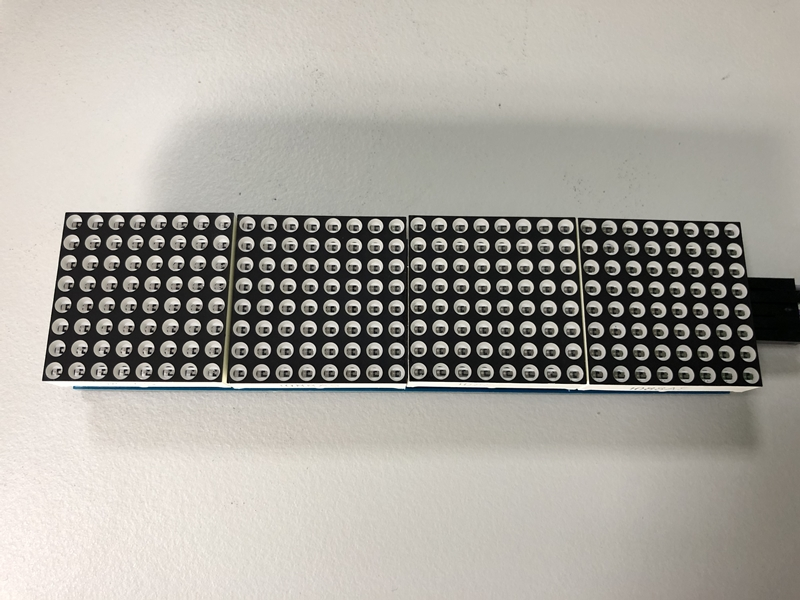# 【NodeMCU】點陣式LED控制LED除了單獨一顆一顆之外，也有排成矩陣方式的以方便我們顯示數字和文字，在這個單元中我們就來看看如何驅動這一類的LED矩陣。

https://github.com/mcauser/micropython-max7219

``````import max7219
import time
messages = ['Nice', ' to ', 'meet', 'you!']
from machine import Pin, SPI
spi = SPI(1, baudrate=10000000, polarity=0, phase=0)
disp = max7219.Matrix8x8(spi, Pin(15), 4)
disp.brightness(0)
while True:
for item in messages:
disp.fill(0)
disp.text(item,0,0,1)
disp.show()
time.sleep(1)``````

``````import max7219
import time
import dht
from ntptime import settime
from machine import Pin, SPI

settime()
d = dht.DHT11(Pin(16))
spi = SPI(1, baudrate=10000000, polarity=0, phase=0)
disp = max7219.Matrix8x8(spi, Pin(15), 4)
disp.brightness(0)
while True:
messages = list()
utc = time.mktime(time.localtime())
year, month, day, hour, minute, second, week, days = time.localtime(utc+28800)
messages.append(str(year))
messages.append("{:2d}{:2d}".format(month, day))
messages.append("{:2d}{:2d}".format(hour, minute))
for item in messages:
disp.fill(0)
disp.text(item,0,0,1)
disp.show()
time.sleep(2)
d.measure()
disp.fill(0)
disp.text('{}C'.format(d.temperature()), 0, 0, 1)
disp.show()
time.sleep(2)
disp.fill(0)
disp.text('{}%'.format(d.humidity()), 0, 0, 1)
disp.show()
time.sleep(2)``````